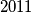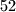IMO Shortlist 2011 problem C7

Kvaliteta:
Avg: 0,0
Težina:
Avg: 9,0
On a square table of$2011$ by$2011$ cells we place a finite number of napkins that each cover a square of$52$ by$52$ cells. In each cell we write the number of napkins covering it, and we record the maximal number$k$ of cells that all contain the same nonzero number. Considering all possible napkin configurations, what is the largest value of$k$?

Proposed by Ilya Bogdanov and Rustem Zhenodarov, Russia
Izvor: Međunarodna matematička olimpijada, shortlist 2011